# Horizontal scaling

Go back to  'Functions'

Suppose that you have the graph of $$y = f\left( x \right)$$. How can you draw the graph of $$y = g\left( x \right) = f\left( {2 x}\right)$$? Suppose that $$\left({{x_0},{y_0}} \right)$$is a point on the curve of the original function.This means that $$f\left( {{x_0}} \right) ={y_0}$$. For what value of x will g output this same value $${y_0}$$? Clearly, it will be $$\frac {{{x_0}}}{2}$$, as the following shows:

$g\left( {\frac{{{x_0}}}{2}} \right) = f\left({2\left( {\frac{{{x_0}}}{2}} \right)} \right) = f\left( {{x_0}} \right) ={y_0}$

This means that a point $$\left( {{x_0},{y_0}} \right)$$ on the curve of  f will correspond to the point $$\left({\frac{{{x_0}}}{2},{y_0}} \right)$$ on the curve of g. Thus, every point on the original graph will come closer to the y-axis by a factor of 2 on the new graph.

In the following figure, the dotted curve corresponds to $$y = f\left( x \right)$$(where f is some function), while the solid curve corresponds to $$y = g\left( x \right) = f\left( {2x} \right)$$: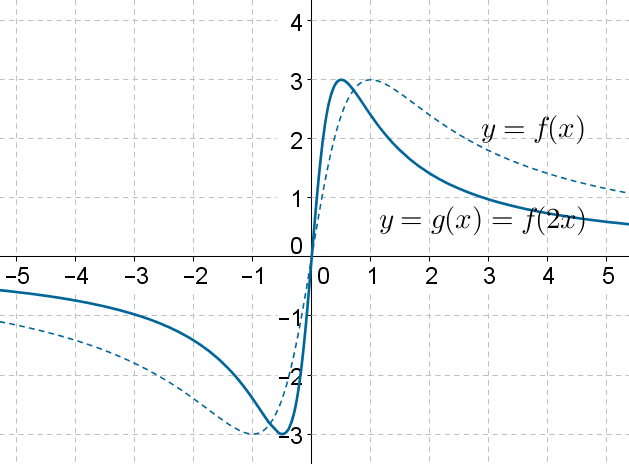Note how the curve has scaled(compressed) horizontally by a factor of 2. For example, the peak at $$\left( {1,3} \right)$$ has shifted to $$\left( {0.5,3} \right)$$ in the new curve.

The following figure shows the graph of $$y = f\left( x \right)$$ and $$y = f\left( {3x} \right)$$ (where f is some function):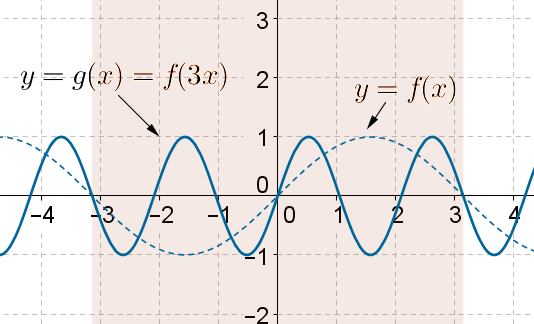Note how the graph of $$y = f\left( {3x} \right)$$ is thrice as fast the graph of $$y = f\left( x \right)$$. In once cycle of the original curve, the new curve completes three cycles(highlighted by the shading). In other words, the graph of $$y = f\left( {3x} \right)$$ can be obtained by horizontally compressing the graph of $$y =f\left( x \right)$$ by a factor of 3.

The following figure shows the graph of $$y = f\left( x \right)$$ and $$y = f\left( {\frac{x}{2}} \right)$$ (where is some function):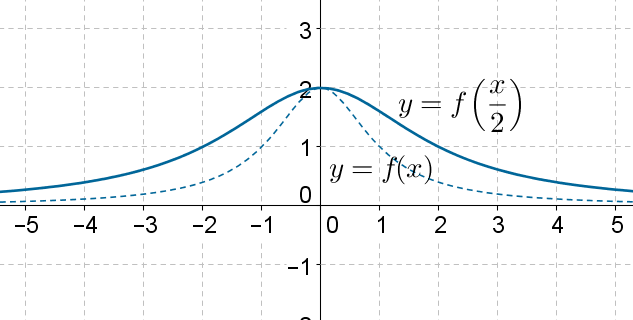Clearly, the graph of $$y = f\left( {\frac{x}{2}} \right)$$ has scaled horizontally by a factor of 2 relative to the graph of $$y = f\left( x \right)$$.

In general, the graph of $$y = f\left( {kx} \right)$$ can be obtained by horizontally scaling the graph of $$y = f\left( x\right)$$ by $$\left| k \right|$$units. If the magnitude of k is greater than 1, then the graph will compress horizontally; if the magnitude of k is less than 1, then the graph will stretch out horizontally. What happens if k is negative? The following figure shows the graph of $$y =f\left( x \right)$$ and $$y = f\left( { -x} \right)$$: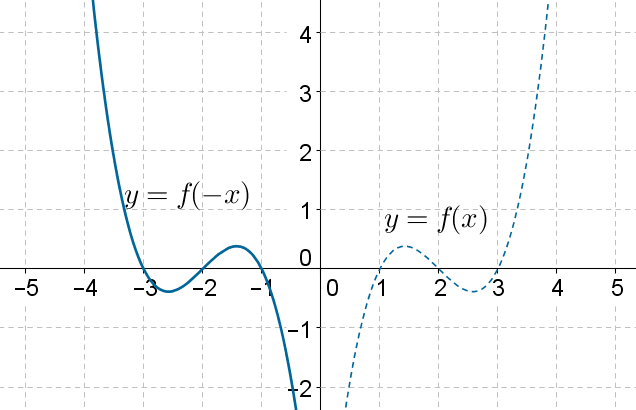The two are mirror images of each other in the y-axis. In other words, the originally curve can be reflected about the y-axis to obtain the new curve. Can you see why?

The following figure shows the graph of $$y = f\left( x \right)$$ and $$y = f\left( { - \frac{x}{2}} \right)$$: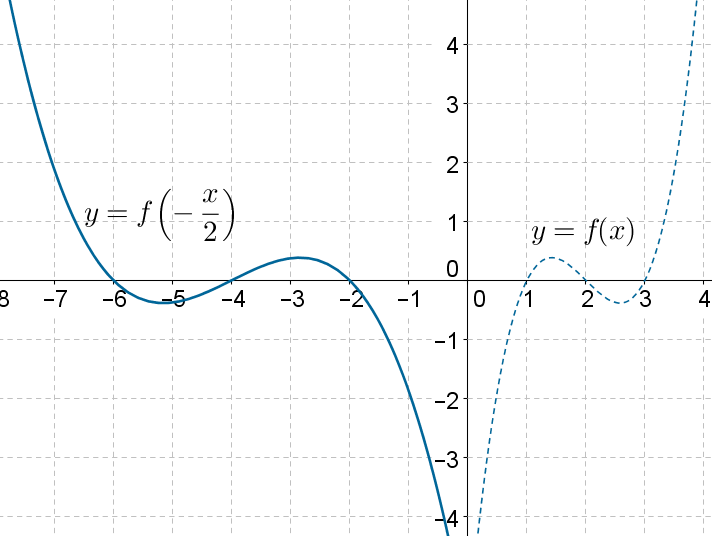This transformation can be thought of as a combination of reflection about the y-axis and then horizontal scaling: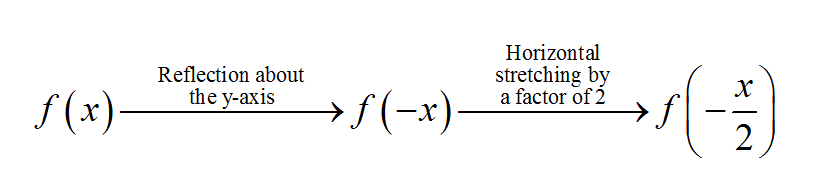Note that in horizontal scaling, the point where the new curve intersects the y-axis is the same as the point where the old curve intersects the y-axis. To see why, suppose that the curve for $$y = f\left( x \right)$$crosses the y-axis at $$\left( {0,{y_0}}\right)$$. This means that $$f\left( 0\right) = {y_0}$$. If the horizontally scaled curve corresponds to $$y = g\left( x \right) = f\left( {kx} \right)$$,then

$g\left( 0 \right) = f\left( {k\left( 0 \right)}\right) = f\left( 0 \right) = {y_0}$

Thus, the curve for g also passes through $$\left( {0,{y_0}} \right)$$.

Functions
Functions
grade 10 | Questions Set 2
Functions
Functions
grade 10 | Questions Set 1